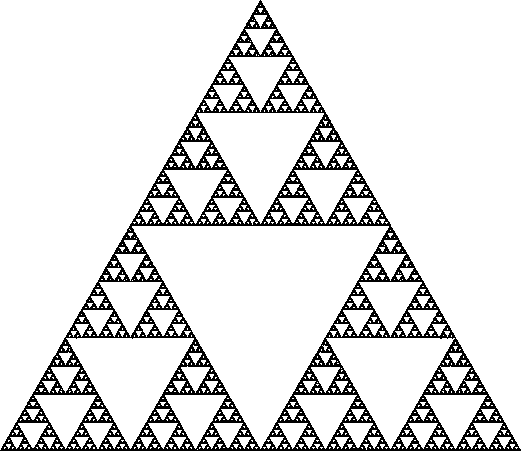# Sierpinski Area

Calculus Level 2This image shows the famous Sierpinski Triangle fractal. If the fractal goes on infinitely, in the limit, what is the area of the black part of this shape as a proportion of the whole triangle?

Assume the image shows a complete Sierpinski triangle.

×